# How to solve (solution) Google's Blockly Future Programmers Game: Bird Level

Google's Blockly Games is a series of educational games that teach programming. It is based on the Blockly library. All code is free and open source. The Bird Game is a deep-dive into conditionals. Control-flow is explored with increasingly complex conditions. The game engine and source is available as an open source project at Github here.

In this article we'll share with you the solution to all 10 levels available in the Bird Game of Blockly.

## Level #1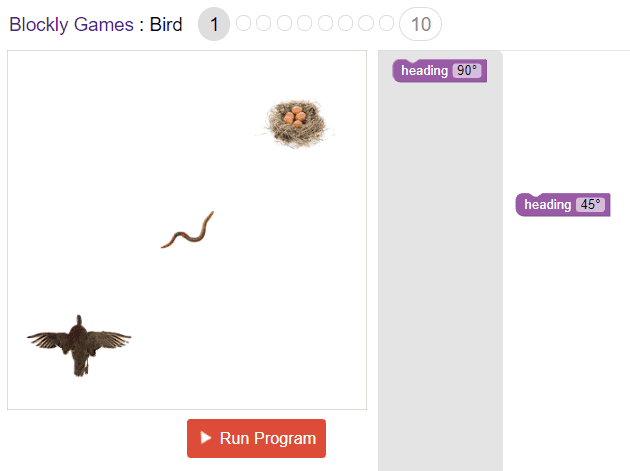The respective JavaScript code of this level is:

``heading(45);``

## Level #2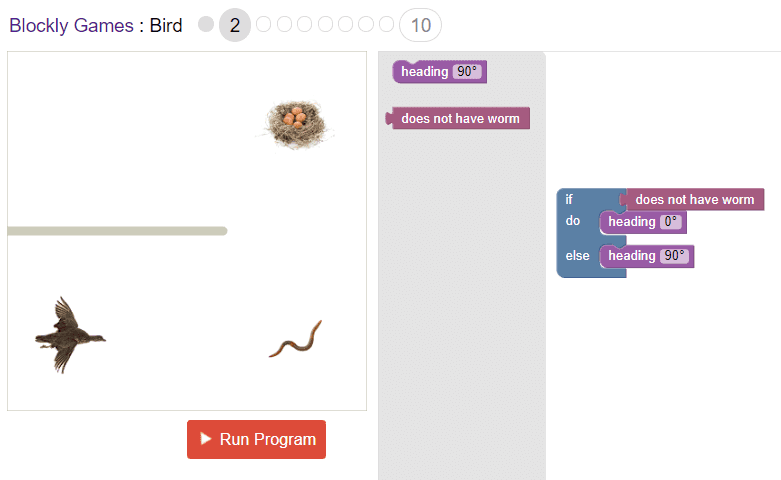The respective JavaScript code of this level is:

``````if (noWorm()) {
} else {
}``````

## Level #3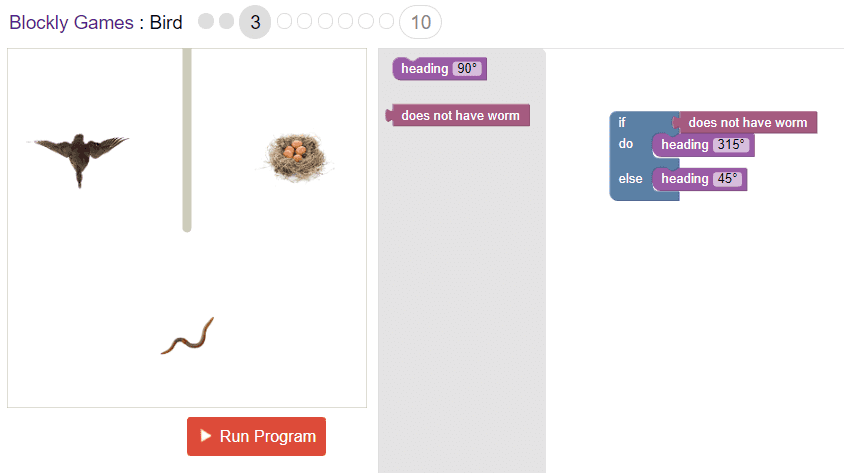The respective JavaScript code of this level is:

``````if (noWorm()) {
} else {
}``````

## Level #4The respective JavaScript code of this level is:

``````if (getX() < 80) {
} else {
}``````

## Level #5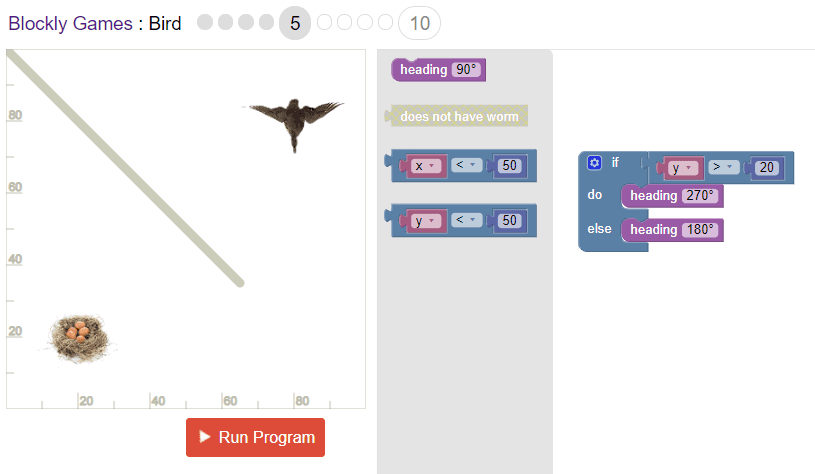The respective JavaScript code of this level is:

``````if (getY() > 20) {
} else {
}``````

## Level #6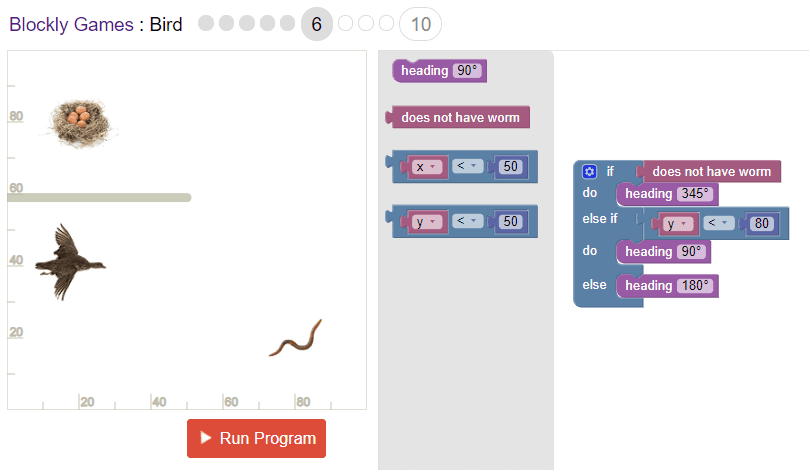The respective JavaScript code of this level is:

``````if (noWorm()) {
} else if (getY() < 80) {
} else {
}``````

## Level #7The respective JavaScript code of this level is:

``````if (getY() > 50) {
} else if (noWorm()) {
} else {
}``````

## Level #8The respective JavaScript code of this level is:

``````if (getY() < 40) {
} else if (noWorm()) {
} else if (getX() > 50 && getY() < 50) {
} else {
}``````

## Level #9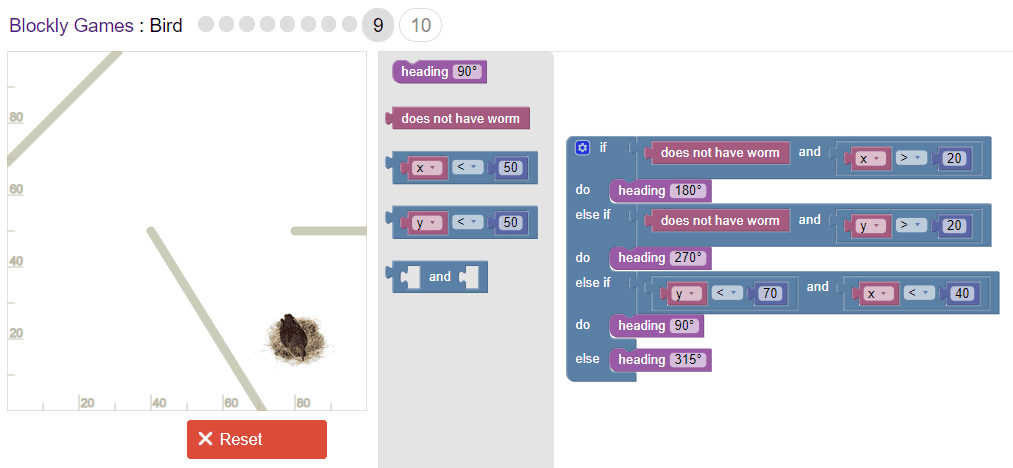The respective JavaScript code of this level is:

``````if (noWorm() && getX() > 20) {
} else if (noWorm() && getY() > 20) {
} else if (getY() < 70 && getX() < 40) {
} else {
}``````

## Level #10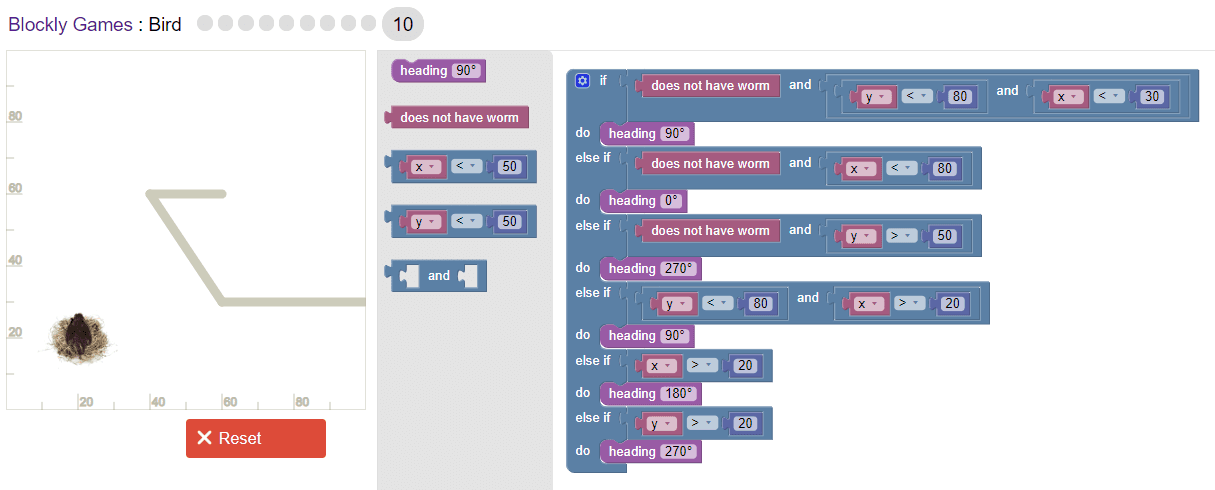The respective JavaScript code of this level is:

``````if (noWorm() && getY() < 80 && getX() < 30) {
} else if (noWorm() && getX() < 80) {
} else if (noWorm() && getY() > 50) {